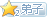# 逍遥兵王混花都

• 手机阅读
• 目录
• 阅读本书

• 赞赏
道具：1
• 送鲜花
鲜花： 1,390
• 投红票
红票： 0

## 我来评论这本书

暂无

### 作者推荐的书

##### 本书粉丝排行 更多
•——粉丝积分： 4,876
•——粉丝积分： 4,268
• 3 大蒋——粉丝积分： 4,036
•——粉丝积分： 3,912
•——粉丝积分： 3,516
•——粉丝积分： 3,468
•——粉丝积分： 3,380
•——粉丝积分： 3,198
•——粉丝积分： 3,196
• 10 李騫——粉丝积分： 3,172
##### 本书动态
• 微信用户823621258对 《逍遥兵王混花都》 进行了收藏
• 微信用户873410323对 《逍遥兵王混花都》 进行了收藏
• QQ用户018917069对 《逍遥兵王混花都》 进行了收藏
• (^з^)#=对 《逍遥兵王混花都》 赠送鲜花10朵
• (^з^)#=对 《逍遥兵王混花都》 赠送鲜花10朵
• (^з^)#=对 《逍遥兵王混花都》 赠送鲜花10朵
• (^з^)#=对 《逍遥兵王混花都》 赠送鲜花10朵
• 18898538对 《逍遥兵王混花都》 进行了收藏
• (^з^)#=对 《逍遥兵王混花都》 赠送鲜花10朵
• (^з^)#=对 《逍遥兵王混花都》 赠送鲜花10朵
• (^з^)#=对 《逍遥兵王混花都》 赠送鲜花10朵
• (^з^)#=对 《逍遥兵王混花都》 赠送鲜花10朵
• (^з^)#=对 《逍遥兵王混花都》 赠送鲜花10朵
• (^з^)#=对 《逍遥兵王混花都》 赠送鲜花10朵
• (^з^)#=对 《逍遥兵王混花都》 赠送鲜花10朵
• (^з^)#=对 《逍遥兵王混花都》 赠送鲜花10朵
• (^з^)#=对 《逍遥兵王混花都》 赠送鲜花10朵
• (^з^)#=对 《逍遥兵王混花都》 赠送鲜花10朵
• (^з^)#=对 《逍遥兵王混花都》 赠送鲜花9朵
• (^з^)#=对 《逍遥兵王混花都》 赠送鲜花9朵
• (^з^)#=对 《逍遥兵王混花都》 赠送鲜花9朵
• (^з^)#=对 《逍遥兵王混花都》 赠送鲜花9朵
• (^з^)#=对 《逍遥兵王混花都》 赠送鲜花9朵
• QQ用户496176028对 《逍遥兵王混花都》 进行了收藏
• (^з^)#=对 《逍遥兵王混花都》 赠送鲜花9朵
• (^з^)#=对 《逍遥兵王混花都》 赠送鲜花9朵
• (^з^)#=对 《逍遥兵王混花都》 赠送鲜花1朵
• (^з^)#=对 《逍遥兵王混花都》 赠送鲜花8朵
• signal对 《逍遥兵王混花都》 进行了收藏
• (^з^)#=对 《逍遥兵王混花都》 赠送鲜花9朵
• (^з^)#=对 《逍遥兵王混花都》 赠送鲜花9朵
• (^з^)#=对 《逍遥兵王混花都》 赠送鲜花9朵
• (^з^)#=对 《逍遥兵王混花都》 赠送鲜花9朵
• (^з^)#=对 《逍遥兵王混花都》 赠送鲜花9朵
• (^з^)#=对 《逍遥兵王混花都》 赠送鲜花9朵
• (^з^)#=对 《逍遥兵王混花都》 赠送鲜花9朵
• (^з^)#=对 《逍遥兵王混花都》 赠送鲜花9朵
• (^з^)#=对 《逍遥兵王混花都》 赠送鲜花9朵
• (^з^)#=对 《逍遥兵王混花都》 赠送鲜花9朵
• (^з^)#=对 《逍遥兵王混花都》 投红票1票
• (^з^)#=对 《逍遥兵王混花都》 赠送鲜花9朵
• (^з^)#=对 《逍遥兵王混花都》 赠送鲜花9朵
• (^з^)#=对 《逍遥兵王混花都》 赠送鲜花9朵
• (^з^)#=对 《逍遥兵王混花都》 赠送鲜花9朵
• (^з^)#=对 《逍遥兵王混花都》 进行了评论
• (^з^)#=对 《逍遥兵王混花都》 进行了评论
• (^з^)#=对 《逍遥兵王混花都》 赠送鲜花9朵
• (^з^)#=对 《逍遥兵王混花都》 赠送鲜花9朵
• (^з^)#=对 《逍遥兵王混花都》 赠送鲜花9朵
• (^з^)#=对 《逍遥兵王混花都》 赠送鲜花9朵
• (^з^)#=对 《逍遥兵王混花都》 进行了评论回复
• (^з^)#=对 《逍遥兵王混花都》 赠送鲜花9朵
• (^з^)#=对 《逍遥兵王混花都》 进行了评论回复
• (^з^)#=对 《逍遥兵王混花都》 赠送鲜花9朵
• (^з^)#=对 《逍遥兵王混花都》 赠送鲜花9朵
• (^з^)#=对 《逍遥兵王混花都》 赠送鲜花9朵
• 傷心孤影对 《逍遥兵王混花都》 进行了收藏
• (^з^)#=对 《逍遥兵王混花都》 赠送鲜花9朵
• (^з^)#=对 《逍遥兵王混花都》 赠送鲜花9朵
• (^з^)#=对 《逍遥兵王混花都》 赠送鲜花9朵
• (^з^)#=对 《逍遥兵王混花都》 赠送鲜花9朵
• (^з^)#=对 《逍遥兵王混花都》 赠送鲜花9朵
• (^з^)#=对 《逍遥兵王混花都》 赠送鲜花9朵
• (^з^)#=对 《逍遥兵王混花都》 赠送鲜花9朵
• (^з^)#=对 《逍遥兵王混花都》 赠送鲜花9朵
• (^з^)#=对 《逍遥兵王混花都》 赠送鲜花9朵
• (^з^)#=对 《逍遥兵王混花都》 赠送鲜花9朵
• (^з^)#=对 《逍遥兵王混花都》 赠送鲜花1朵
• (^з^)#=对 《逍遥兵王混花都》 赠送鲜花8朵
• (^з^)#=对 《逍遥兵王混花都》 进行了评论回复
• (^з^)#=对 《逍遥兵王混花都》 赠送鲜花8朵
• (^з^)#=对 《逍遥兵王混花都》 投红票1票
• (^з^)#=对 《逍遥兵王混花都》 赠送鲜花8朵
• (^з^)#=对 《逍遥兵王混花都》 进行了评论
• (^з^)#=对 《逍遥兵王混花都》 赠送鲜花8朵
• (^з^)#=对 《逍遥兵王混花都》 赠送鲜花8朵
• (^з^)#=对 《逍遥兵王混花都》 赠送鲜花8朵
• (^з^)#=对 《逍遥兵王混花都》 赠送鲜花8朵
• (^з^)#=对 《逍遥兵王混花都》 赠送鲜花8朵
• (^з^)#=对 《逍遥兵王混花都》 赠送鲜花8朵
• (^з^)#=对 《逍遥兵王混花都》 赠送鲜花8朵
• (^з^)#=对 《逍遥兵王混花都》 赠送鲜花8朵
• (^з^)#=对 《逍遥兵王混花都》 赠送鲜花8朵
• (^з^)#=对 《逍遥兵王混花都》 赠送鲜花8朵
• (^з^)#=对 《逍遥兵王混花都》 赠送鲜花8朵
• (^з^)#=对 《逍遥兵王混花都》 赠送鲜花8朵
• (^з^)#=对 《逍遥兵王混花都》 赠送鲜花8朵
• (^з^)#=对 《逍遥兵王混花都》 赠送鲜花8朵
##### 版权说明：

《逍遥兵王混花都》由作者 花落成泥 于 2016-11-30 创建并上传，本站仅提供网络存储空间，本作品与本站立场无关。